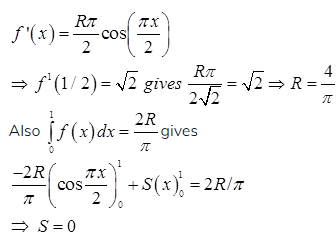# GATE Solved Paper 2017-19 - GATE 2017 Shift 2

>>>>>>>>GATE 2017 Shift 2

• A

P-(iii), Q-(iv), R-(i), S-(ii)• B

P-(iv), Q-(iii), R-(i), S-(ii)• C

P-(iii), Q-(iv), R-(ii), S-(i)• D

P-(iv), Q-(iii), R-(ii), S-(i)• Option : C
• Explanation :
P. Towers of Hanoi
⇒ T(n) = 2T(n-1) + 1 ⇒ θ(2n)
Q. Binary search
⇒ T(n) = T(n/2) + c ⇒ θ(log n)
R. Heap sort ⇒ θ(nlog n)
S. Addition of two n × n matrices ⇒ θ(nr)

• A

P-(ii), Q-(iii), R-(iv), S-(i)• B

P-(ii), Q-(i), R-(iii), S-(iv)• C

P-(iii), Q-(iv), R-(i), S-(ii)• D

P-(i), Q-(iv), R-(ii), S-(iii)• Option : C
• Explanation :
Lexical Analysis phase processes character stream and generates tokens, e.g. identifier or keywords.
Tokens are processed by Syntax analysis analyzer.
Syntax tree is processed by Semantic analyzer.
Intermediate code such as 3 –address code is used for code generation process.

• A

I and IV only• B

I, II and III only• C

I, II and IV only• D

II, III and IV only• Option : C
• Explanation :
Statement (1): RIP uses distance vector routing. “CORRECT”
RIP is one of the oldest DVR protocol which employ the hop count as a routing metric.
Statement (2): RIP packets are sent using UDP. “CORRECT”
RIP uses the UDP as its transport protocol, and is assigned the reserved port no 520
Statement (3): OSPF packets are sent using TCP. “INCORRECT”
OSPF does not use a transport protocol, such as UDP (or) TCP, but encapsulates its data directly in IP packets.
Statement (4): OSPF operation is based on link state routing. “CORRECT”
OSPF is a routing protocol which uses link state routing (LSR) and works within a single autonomous system.
Hence Option “C” is correct.

• Option : D
• Explanation :• A

I and III only• B

II only• C

III only• D

II and III only• Option : D
• Explanation :
Contiguous allocations suffer from external fragmentation. But linked and indexed allocation schemes free from external fragmentation. Hence, option D is correct.
Related Quiz.
GATE 2017 Shift 2